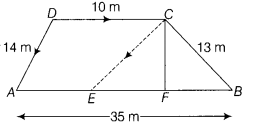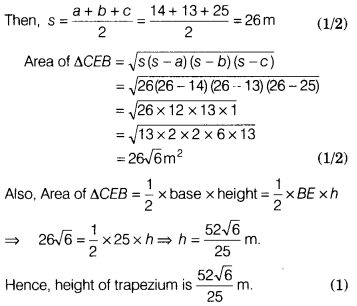# A field is in the shape of a trapezium whose parallel sides are 35 m and 10 m

A field is in the shape of a trapezium whose parallel sides are 35 m and 10 m. The non-parallel sides are 14 m and 13 m. Find the height of the trapezium.

Let ABCD be the given field in the form of trapezium in which AB = 35 m, CD = 10 m, BC = 13 m, AD = 14 m, and DC II ABThrough C, draw CE || DA and let it meet AB at E.
Let h metres (CP) be the height of the trapezium.
DC || AE and CE|| DA
∴ AECD is a parallelogram.
AE = DC = 10 m
and CE = DA = 14 m
ln ∆ CEB, CB = 13m,CE = 14m
and BE = AB - AE = 35 -10 = 25 m
Let a = 14m,b = 13m and c = 25 m